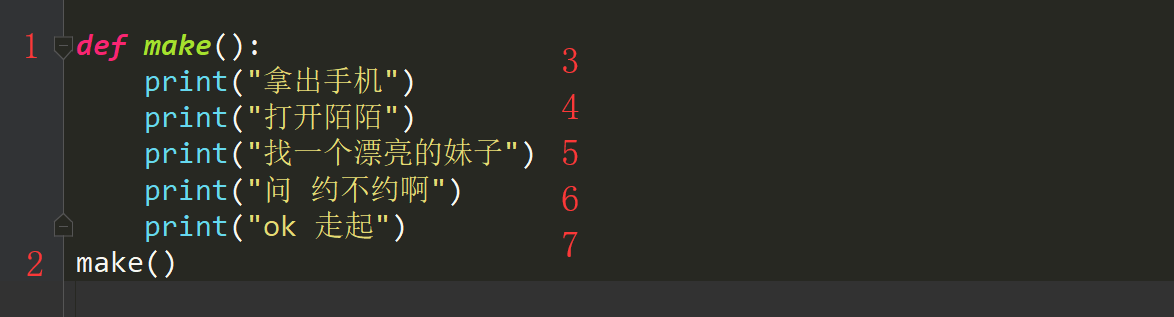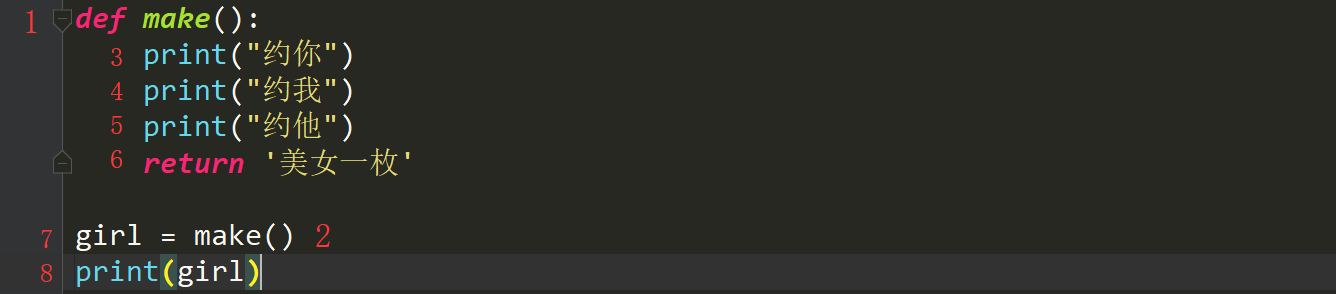# 万恶之源 - Python初识函数

### 什么是函数

pint("拿出手机")
print("打开陌陌")
print("找个漂亮的妹子")
print("问她,约不约啊!")
print("ok 走起")

ok so easy我们已经完成了对一个功能的描述,那么问题来了,我还想在约一次怎么搞,是不是就是在写一遍

# 约一次
pint("拿出手机")
print("打开陌陌")
print("找个漂亮的妹子")
print("问她,约不约啊!")
print("ok 走起")

# 再约一次
pint("拿出手机")
print("打开陌陌")
print("找个漂亮的妹子")
print("问她,约不约啊!")
print("ok 走起")

ok 非常简单搞定了,但是我还想约上个10次8次的,怎么搞,加个循环不就行了吗?

while True:
pint("拿出手机")
print("打开陌陌")
print("找个漂亮的妹子")
print("问她,约不约啊!")
print("ok 走起")

### 函数的定义

def 函数名():
函数体

def make():
print("拿出手机")
print("打开陌陌")
print("找一个漂亮的妹子")
print("问她,约不约啊")
print("ok 走起")


### 函数的调用

# 调用yue函数

def make():
print("拿出手机")
print("打开陌陌")
print("找一个漂亮的妹子")
print("问 约不约啊")
print("ok 走起")

make()   # 这是make函数的调用

ok. 走你

### 函数的执行流程make()
make()
make()
make()
make()
make()

### 函数的返回值

def make():
print("约你")
print("约我")
print("约他")
return
print("约谁呀")    # 这句话不会被执行

make()


def make():
print("约你")
print("约我")
print("约他")
return  "美女一枚"

make()

def make():
print("约你")
print("约我")
print("约他")
return '美女一枚'

girl = make()
print(girl)1.定义了一个函数make

2.调用函数

3.执行函数里的约你

4.执行函数里的约我

5.执行函数里的约他

6.返回给调用者一个字符串'美女一枚',这个调用者就是make()

7.将返回的字符串通过一个等号赋值给白变量girl

8.打印变量girl的值

def make():
print("约你")
print("约我")
print("约他")
return "美女一枚", "萝莉一枚"
girl = make()
print(type(girl))   # tuple

1.遇到return,此函数结束,函数后面的东西将不会在执行

2.return 返回值

关于返回值:

如果return什么都不写或者干脆就没写return,返回的结果就是None

如果return后面写了一哥值,则调用者可以接受一个结果

如果return后面写了多个结果,则调用者可以接受一个tuple,调用者可以直接使用解构获取多个变量

OK,完美 可以得到结果了 但是我们约的方式是不是有点问题啊?陌陌现在还能约到吗?约不到了 我们换探探 那要是过几天探探也不行了

### 函数的参数

def 函数名(参数):
函数体　　

def make(chat):
print("拿出⼿手机")
print("打开"+chat)
print("找个漂亮的妹⼦子")
print("约不不约")

make("陌陌")
make("微信")
make("探探")

### 参数

1.形参

写在函数声明的位置的变量叫形参,形式上的一个完整.表示这个函数需要xxx

2.实参

在函数调用的时候给函数传递的值.加实参,实际执行的时候给函数传递的信息.表示给函数xxx

3.传参

给函数传递信息的时候将实际参数交给形式参数的过程被称为传参.

def make(chat):    # chat  形参
print("拿出手机")
print("打开"+chat)
print("找个漂亮的妹子")
print("约不约")

make("陌陌")    # 实参
len("字符串")    # "字符串"在这⾥里里就是实参
print("麻花藤")    # "麻花藤"就是实参　　

#### 参数的分类

1.位置参数

约到现在,有没有想过这么一个问题,什么样的都约么? 哪里的都约么? 不一定吧.比如我现在在北京,我很寂寞,我喜欢小姐姐

alex在泰国,很寂寞 来一个就行了 .需要是不一样的 而我们现在的函数没没有这写功能 那怎么办呢? 很简单 多来几个参数就好了

def make(chat,addr,age):    # chat  形参
print("拿出手机")
print("打开"+chat)
print("找个" + addr +"附近漂亮的" + str(age) + "岁妹子")
print("约不约")

make("陌陌","北京",18)    # 实参



def f(a,b):    c = a+b    return cnum_sum = f(5,8)print(num_sum)
结果: 13

def f(a,b):
if a>b:
return a
else:
return b

num_sum = f(5,8)
print(num_sum)



def f(a,b):
c = a if a > b else b  #当a>b就把a赋值给c,否则就把b赋值给c
return c
msg = f(5,7)
print(msg)

7

def make(chat, address, age):
print("拿出手机")
print("打开"+chat)
print("约不约")

make(chat="微信", age=18, address="北京")       # 关键字参数.

# 混合参数 make("微信", age=18, address="上海")# 正确.第一个位置赋值给chat, 后面的参数开始指定关键字.
make(age="18", "微信", address="广州")    # 错误, 最开始使用了关键字参数, 那么后面的 微信的位置就串了, 容易出现混乱

1. 位置参数

2. 关键字参数

3. 混合参数,  位置参数必须在关键字参数前面

位置参数,按照位置来赋值,到目前为止,我们编写的函数都是这种

def make(chat, address, age):
print("拿出手机")
print("打开"+chat)
print("约不约")


在函数声明的时候, 就可以给出函数参数的默认值. 在调用的时候可以 给出具体的值, 也可以不给值, 使⽤用默认值. 比如, 我们录入咱们班学生的基本信息. 通过调查发现. 我们班大部分学生都是男生. 这个时 候就可以给出⼀一个sex='男'的默认值.

def stu_info(name, age, sex='男'):
print("录入学生信息")
print(name, age, sex)
print("录入完毕")

stu_info("张强", 18)

综上:在形参的角度来看

1. 位置参数

2. 默认值参数(大多数传进来的参数都是一样的, 一般用默认参数)

1.位置参数 就是按照顺序传递

2.默认值参数(在填写参数的时候需要指定传递到形参中那个变量)

posted @ 2018-10-11 19:30 Meets 阅读(...) 评论(...) 编辑 收藏